Mobile QR Code1. (School of Electronic and Electrical Engineering, Hankyong National University, Anseong 17579, Korea )
2. (School of Electrical Engineering, Pukyong National University, Busan 48513, Korea )
3. (Department of Electrical Engineering, Inha University, Incheon 22212, Korea)

Instability mechanism in α-HIZO TFT, temperature effects on electrical properties in α-HIZO TFT

## I. INTRODUCTION

Recently, amorphous hafnium-indium-zinc oxide (α-HIZO) thin film transistors (TFTs) have received lots of attention due to many advantages such as transparency, high mobility (> 10 cm$^{2}$/V·sec), a good uniformity, possibility of low-temperature process, and excellent bias-stress stability (1-6). Despite these advantages, α-HIZO TFTs suffer from the instability problems induced by various stresses such as bias and light which is similar to the real circumstance. Therefore, numerous researching groups have studied on the instability under light and/or dc-bias stress (7-10). Especially, threshold voltage (V$_{TH}$) shift and hump occurrence after the stresses have been considered as one of the most critical issues in real application. Thus, in our group, the cause of these abnormal behaviors by various stresses has been analyzed and revealed to improve the electrical instability of α-HIZO TFTs (11-13).

In this work, the hump characteristics generated by light irradiation and dc-bias stresses are investigated in terms of the hump current components as well as the physical mechanism. To figure out the current flowing mechanism, rigorously, the transfer characteristics and source-to-drain currents with floated gate (S/D current) of α-HIZO TFTs are explored at various temperatures. Moreover, to understand the origin of the hump current, the changes of the S/D current by stress are experimentally evaluated and analyzed through TCAD simulations.

## II. Device Fabrication

Fig. 1. (a) Schematic diagrams of bottom gate TFT structure, (b) Cross-sectional TEM image of the fabricated α-HIZO TFT along the channel length direction.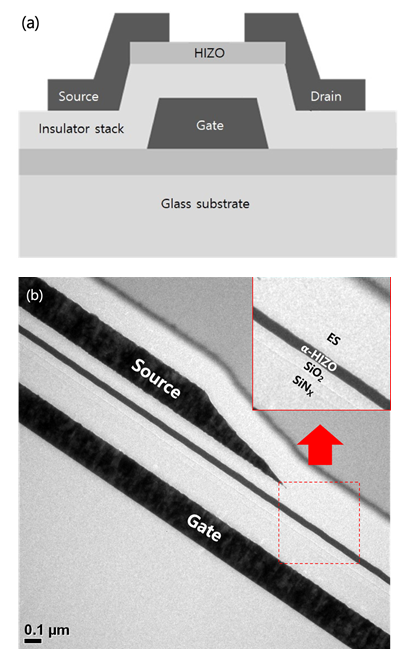Fig. 2. I$_{D}$-V$_{G}$ characteristic after applying the negative gate bias of -20 V for 1000 s with the light irradiation.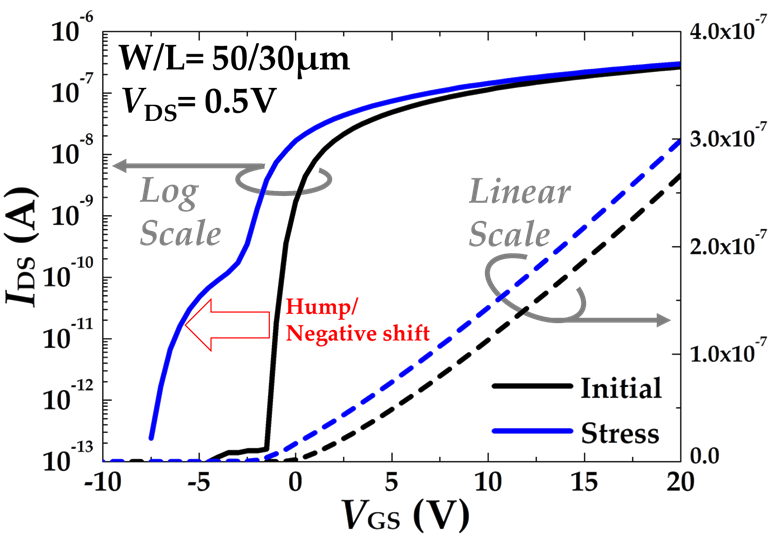The cross-sectional schematic diagram for the fabricated oxide TFT is shown in Fig. 1(a). The fabricated device has an inverted staggered structure. On a glass wafer, the gate is formed with molybdenum. The gate insulators are deposited as a bi-layer consisting of 250-nm thick SiN$_{x}$ and 50-nm thick SiO$_{2}$, where the SiN$_{x}$ layer is contacted with the gate electrode. Active α-HIZO with thickness of 40 nm is formed by radio-frequency (RF) sputtering on the gate insulator at room temperature, which consists of In, Zn, O, and Hf. The 100-nm thick protective SiO$_{2}$ layer is deposited to prevent the active α-HIZO from etching damage during S/D wet etching process. Then, the S/D region is sputtered with 200-nm thick molybdenum on the active, followed by S/D wet etching. For a passivation, the 200-nm SiO$_{2}$ film is grown. Finally, the devices are annealed in the N$_{2}$ ambient for 5 h at 250 $^{\circ}$C. The cross-sectional transmission electron microscopy (TEM) image for the fabricated α-HIZO TFT is shown in Fig. 1(b).

## III. Experimental Results and Discussions

The electrical characteristics of the α-HIZO TFTs are analyzed by using HP4156 precision semiconductor parameter analyzer and white LED for optical stresses.

In order to reproduce the effects of the practical stress for display operating conditions, the -20 V of gate bias is applied to the gate electrode under an optical stress of 8000 cd/m$^{2}$ for 1000 s using a white LED. As can be seen in Fig. 2, two significant phenomena are observed after the simultaneous application of light and negative gate bias: 1) the hump occurrence; and 2) the V$_{TH}$ shift to the negative direction. These can be explained by the fact that photo-generated holes in n-type channel are trapped in the gate insulator by the e-field enhanced by the optical energy (12). Also, it is experimentally revealed that the hump gets induced by the particularly trapped holes in the gate insulator at the edge regions along the channel width direction (12). In other words, the current path starts to be formed from the edge regions since the trapped holes can modulate the energy band of the TFT.

For the better understanding on this stress-induced current flowing, the conduction mechanism of α-HIZO TFTs should be clarified. Hence, the I$_{D}$-V$_{G}$ characteristics with temperature variations (273 K, 293 K, 313 K, and 333 K) are investigated with V$_{D}$ = 0.5 V in the dark as shown in Fig. 3(a). As temperature goes up, the V$_{TH}$ gets reduced, and the subthreshold swing (SS}) becomes degraded as similar to the conventional silicon transistors. Fig. 3(b) describes the detailed SS and the on-current with respect to temperature, respectively. Interestingly, it is observed that the on-current is gradually enhanced with the temperature increase. This phenomenon is totally different from the conventional one which has the on-current reduction by temperature increases due to mobility degradation (14).

Fig. 3. (a) I$_{D}$-V$_{G}$ characteristics measured at various temperatures (273 K, 293 K, 313 K, and 333 K), (b) Temperature dependence on the subthreshold swing (SS}), and on-current.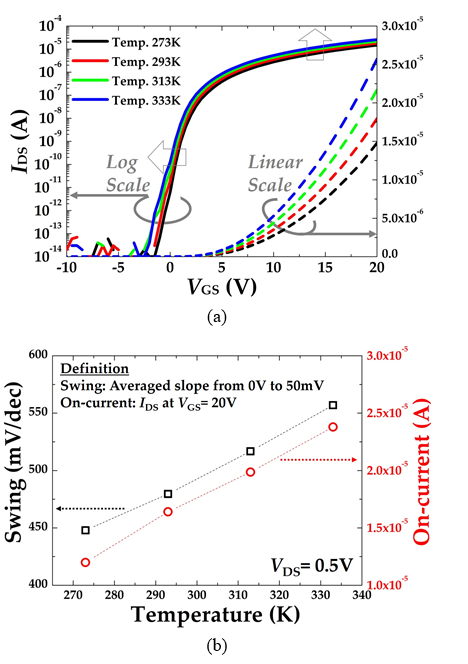Fig. 4. I$_{D}$-V$_{D}$ characteristics to characterize the J$_{TFE}$ dominant region under a large reverse bias. Inset shows the I$_{DS}$-V$_{DS}$$^{1/2}$ characteristics for the J$_{gen}$ dominant region under a low reverse bias.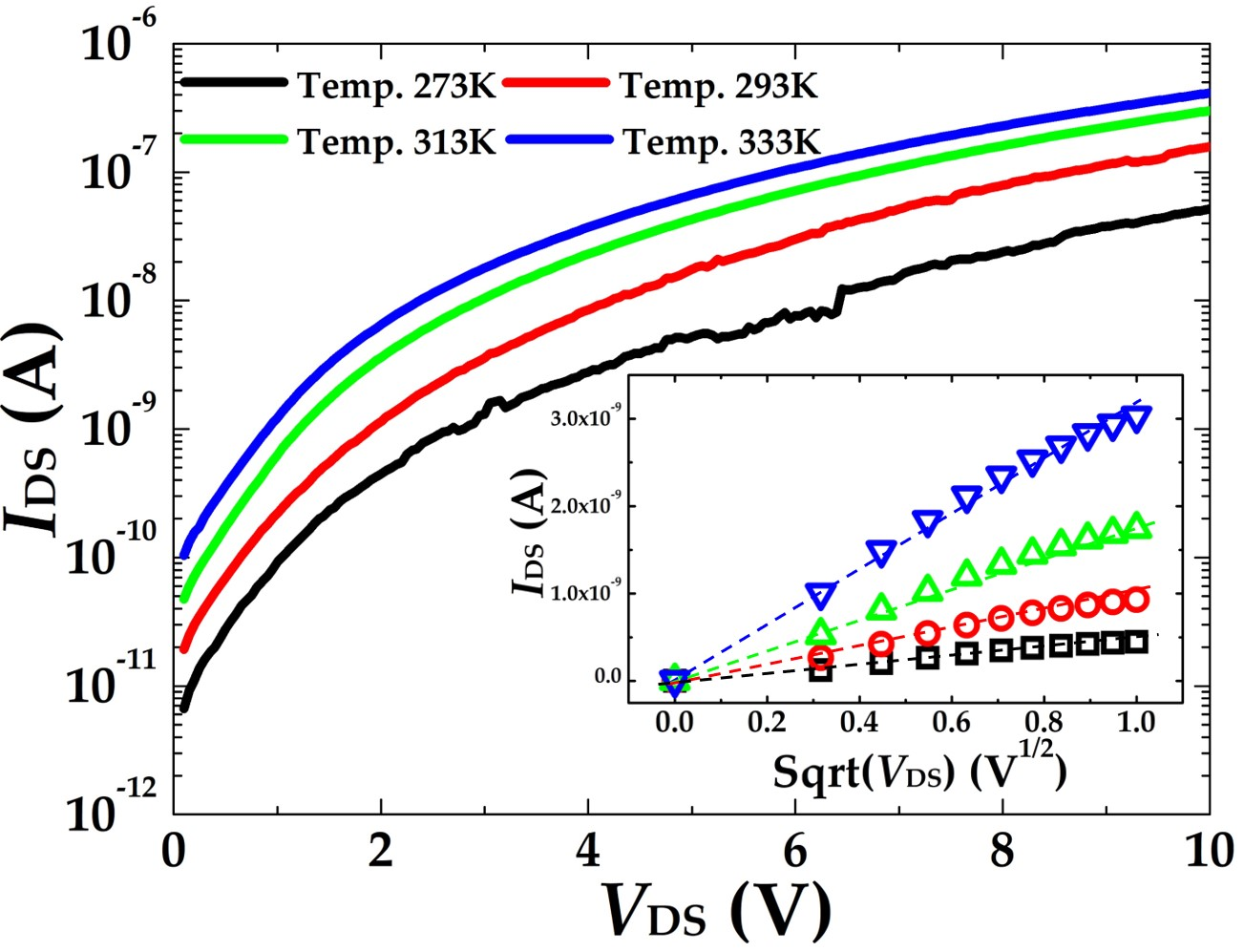In order to understand the origin of the distinguished temperature dependence in the α-HIZO TFT, the S/D current is measured with grounded source by sweeping V$_{D}$ at various temperatures. Fig. 4 indicates that the S/D current (I$_{D}$-V$_{D}$ characteristics) gets increased with the elevated temperatures. This phenomenon can be understood as follows. Considering the increase of the on-current with the higher temperature, it is expected that the S/D metal contacted with the active α-HIZO forms the Schottky barrier diode (SBD) (15). Furthermore, the α-HIZO TFTs can be modeled as two oppositely connected SBDs with a resistor between them, which are modulated by V$_{G}$ (16-18). Thus, the S/D current is suppressed by the reverse biased Schottky contact and the main current components consist of thermionic emission (J$_{TE}$), thermionic field emission (J$_{TFE}$), and thermal generation (J$_{gen}$) currents (19). Notably, the trap-assisted generation current (J$_{gen}$) in the space charge region (SCR) is dominantly observed under a low V$_{D}$ since the S/D current is proportional to (1+ V$_{R,Schottky}$ / V$_{bi}$)$^{1/2}$ [inset of Fig. 4], whereas the thermionic field emission current (J$_{TFE}$) is mainly generated at a large reverse bias because the S/D current is proportional to exp(qV$_{R,Schottky}$ / E). Here, V$_{R,Schottky}$, V$_{bI}$, and E are the voltage across reverse-biased Schottky diode, the built-in voltage, and the characteristic energy for the thermionic field emission current, respectively. Therefore, it is confirmed that the significant increases of J$_{TE}$ and J$_{TFE}$ with the higher temperatures in the reverse-biased Schottky contact cause the V$_{TH}$ shift, the SS degradation, and the on-current enhancement like a Schottky barrier FET.

Subsequently, the change of the S/D current by stresses is investigated at room temperature by considering the reverse-biased Schottky diode characteristics. To simplify the analysis, the -20 V of bias is applied to the gate and drain electrodes with the grounded source under the light irradiation to trap the photo-generated holes in the gate insulator only at the source-side edge region. As shown in Fig. 5, it is confirmed that the S/D current becomes increased after applying the stress as compared to the initial state. This implies that the trapped holes by the stress affects the source-side Schottky barrier, leading to the S/D current enhancement.

Fig. 5. I$_{D}$-V$_{D}$ characteristics between source and drain with floated gate after the stress (V$_{G}$ and V$_{D}$ = -20 V with the source grounded under light irradiation). It is to confirm the impacts of the trapped holes on the tunneling barrier in the identical TFT.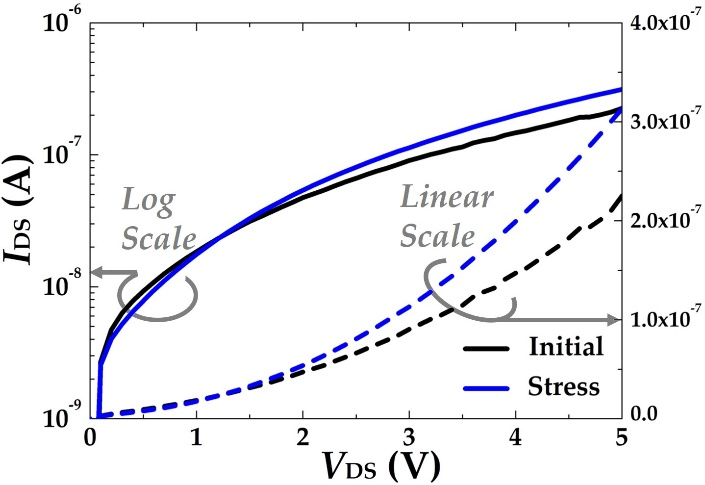Fig. 6. (a) Simulated device structure and location of the trapped holes uniformly inserted in the gate insulator, (b) Simulated conduction band diagram of the SBD formed between the source and the channel (A-A’). The dependence of the tunneling barrier on the trapped holes was simulated.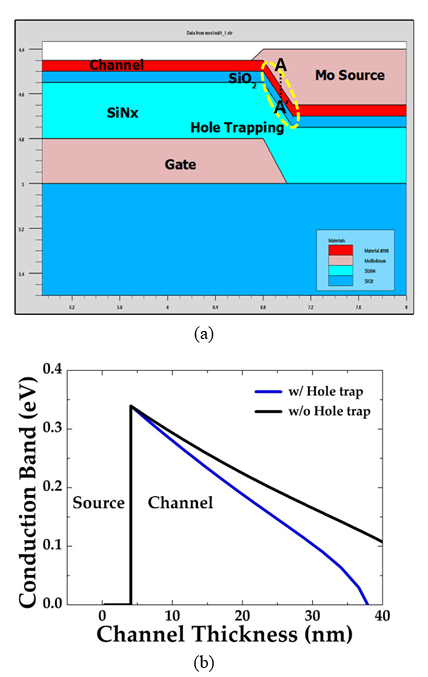Moreover, to verify the current enhancement by the trapped holes, TCAD device simulations are performed using SILVACO$^{TM}$ ATLAS (20). In order to implement Schottky barrier characteristics at the interface between S/D and α-HIZO channel, universal Schottky tunneling (UST) and surface recombination models are used to consider tunneling and thermionic emission components with fermi distribution. Based on the same experimental materials and dimensions, holes are locally defined at the SiO$_{2}$/α-HIZO channel interface of the edge region along the channel width direction to reflect the stress effects (V$_{G}$ = -20 V with the grounded S/D under light irradiation) [Fig. 6(a)]. Then, to confirm the effects of the trapped holes on electrical characteristics, the energy band diagrams are extracted (dashed yellow region) with/without the trapped holes as shown in Fig. 6(b). It is clearly found that the Schottky barrier width between the source and the α-HIZO channel becomes thinner by the trapped holes while the Schottky barrier height remains almost unchanged. Consequently, the trapped holes located at the edge regions make the enhancement of the S/D current by increasing J$_{gen}$ and J$_{TFE}$(thinner Schottky barrier width) without the help of J$_{TE}$ (unchanged Schottky barrier height), which is consistent with the results of Fig. 4.

## IV. CONCLUSIONS

In this work, the hump characteristics caused by bias and optical stresses are analyzed through the electrical measurements and TCAD simulations. To verify the physical origin of the hump current, the transfer characteristics and the S/D current are investigated with temperature variations before the stress and then the change of the S/D current by the simultaneous bias and optical stresses is measured. As a result, it is confirmed that the S/D metals contacted with the active α-HIZO form the oppositely connected SBDs. Also, it is revealed that the S/D current enhancement after applying the stresses can be understood that the trapped holes make the Schottky barrier narrowing near the source-side edge regions and the hump occurs by the increase of J$_{gen}$ and J$_{TFE}$.

### ACKNOWLEDGMENTS

This research was supported in part by INHA UNIVERSITY Research Grant, in part by the Future Semiconductor Device Technology Development Program (20010847) funded by the Ministry of Trade, Industry and Energy (MOTIE) and the Korea Semiconductor Research Consortium (KSRC); and in part by the National Research Foundation (NRF) funded by the Korean Ministry of Science and ICT under Grant Nos. 2020M3F3A2A01081670, 2020R1G1A1101263, and 2020R1A2C2103059. The EDA tool was supported by the IC Design Education Center (IDEC), Korea.

### REFERENCES

1
Lee J. H., Nam W. ., Kim B. K., Choi H. S., Ha Y. M., Han M. K., 2006, A new poly-Si TFT current-mirror pixel for active matrix organic light emitting diode, IEEE Electron Device Letters, Vol. 27, No. 10, pp. 830-8332
Goncalves G., Barquinha P., Raniero L., Martins R., Fortunato E., 2008, Crystallization of amorphous indium zinc oxide thin films produced by radio-frequency magnetron sputtering, Thin Solid Films}, Vol. 516, No. 7, pp. 1374-13763
Kim C., Dec. 2012, Amorphous hafnium-indium-zinc oxide semiconductor thin film transistors Amorphous hafnium-indium-zinc oxide semiconductor thin film transistors, Appl. Phys. Lett., Vol. 252103, No. 2009, pp. 1-44
Gao Y., Li X., Chen L., Shi J., Sun X.W., Zhang J., 2014, High Mobility Solution-Processed Hafnium Indium Zinc Oxide TFT With an Al-Doped ZrO2 Gate Dielectric, IEEE Electron Device Letters, Vol. 35, No. 5, pp. 554-5565
Kim J. H., Chang J., Kim S., Park B.-G., 2019, Oxide Thin Film Transistor With a Novel Gate Insulator Stack to Suppress Photo-Excited Charge Injection, IEEE Transactions on Nanotechnology, Vol. 18, pp. 491-4936
Abliz A., 2020, Investigation of the electrical properties and stability of HfInZnO thin-film transistors, Chinese Journal of Physics, Vol. 68, pp. 788-7957
Chong E., Jo K. C., Lee S. Y., Apr. 2010., High stability of amorphous hafnium-indium-zinc-oxide thin film transistor, Applied Physics Letters, Vol. 96, No. 15, pp. 1521028
Park J. S., Jun. 2010., The influence of SiOx and SiNx passivation on the negative bias stability of Hf-In-Zn-O thin film transistors under illumination, Applied Physics Letters, Vol. 96, No. 26, pp. 2621099
Park J. S., 2010, Influence of illumination on the negative-bias stability of transparent hafniumindiumzinc oxide thin-film transistors, IEEE Electron Device Letters, Vol. 31, No. 5, pp. 440-44210
Shin J. H., Feb. 2009, Light effects on the bias stability of transparent ZnO thin film transistors, ETRI Journal, Vol. 31, No. 1, pp. 62-6311
Kwon D. W., 2010, Charge injection from gate electrode by simultaneous stress of optical and electrical biases in HfInZnO amorphous oxide thin film transistor, Applied Physics Letters, Vol. 97, No. 1912
Kim J. H., 2011, Investigation on the characteristics of stress-induced hump in amorphous oxide thin film transistors, Applied Physics Letters, Vol. 99, No. 413
Kwon D. W., Kim J. H., Park B. G., 2017, The effect of drain bias stress on the instability of turned-OFF amorphous HfInZnO thin-film transistors under light irradiation, IEEE Transactions on Electron Devices, Vol. 64, No. 1, pp. 153-15814
Andricciola P., Tuinhout H. P., 2009, The temperature dependence of mismatch in deep-submicrometer bulk MOSFETs, IEEE Electron Device Letters, Vol. 30, No. 6, pp. 690-69215
Song I., 2008, Short channel characteristics of gallium-indium-zinc-oxide thin film transistors for three-dimensional stacking memory, IEEE Electron Device Letters, Vol. 29, No. 6, pp. 549-55216
Larson J. M., Snyder J. P., 2006, Overview and status of metal S/D Schottky-barrier MOSFET technology, IEEE Transactions on Electron Devices, Vol. 53, No. 5, pp. 1048-105817
Zhu S., 2011, CMOS technology: Schottky-barrier S/D MOSFETs with high-K gate dielectrics and metal-gate electrode, Selected Semiconductor Research, Vol. 25, No. 5, pp. 330-33218
Zhu S., 2004, N-type Schottky barrier source/drain MOSFET using Ytterbium silicide, IEEE Electron Device Letters, Vol. 25, No. 8, pp. 565-56719
Lee S., Mar. 2010., Modeling and characterization of metal-semiconductor-metal-based source-drain contacts in amorphous InGaZnO thin film transistors, Applied Physics Letters, Vol. 96, No. 11, pp. 11350620
Silvaco Inc. , 2016, Atlas User’s Manual, Silvaco Inc.## Author

received the B.S. degree from the Kyungpook National University (KNU), Daegu, in 2008, and the M.S. and Ph.D. degrees in Electrical Engineering from Seoul National University (SNU), Seoul, South Korea, in 2010 and 2015, respectively.

From 2015 to 2021, he was a Senior Engineer with Samsung Electronics, Hwaseong, South Korea.

He is currently an Assistant Professor with the School of Electronic and Electrical Engineering, Hankyong National University, Anseong, South Korea.

His current interests for research include low operating power CMOS devices, nano fabrication developments, and ferroelectric memory applications.

received the B.S. degree in the Electrical Engineering from Korea Advanced Institute of Science and Technology (KAIST), in 2009 and received the M.S., Ph.D. degrees in the Department of Elec-trical Engineering from Seoul Na-tional University, Seoul, Korea, in 2011, and 2016, respectively.

From 2016 to 2020, he worked as a senior researcher at SK hynix. He has working as an assistant professor in the Pukyong National University, Korea.

His interests include low power CMOS, Oxide TFT and Power semiconductors.

received Ph.D. degrees in Electrical Engineering at Seoul National University (SNU), Seoul, Korea, in 2017.

He was a senior engineer at Samsung Electroics from 2005 to 2014.

He was with University of California at Berkeley, USA, in 2017, as a Post-Doctoral Fellow and with Intel, CA, USA, in 2019.

He is currently an Assistant Professor with the Department of Electric Engineering, Inha University, Incheon, Korea.